# High School Chemistry : Calculating Error

## Example Questions

2 Next →

### Example Question #71 : High School Chemistry

A student found out through experimentation that it requires 23.6 mL of a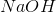solution to completely neutralize 25 mL of a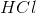solution. However, the actual amount ofsolution needed to neutralize 25 mL of thesolution is 22.8 mL. What is the percent error?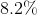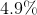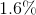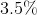Explanation:

Use the following formula to find the percent error: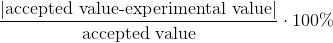For this experiment, our accepted value is the same as the theoretical value.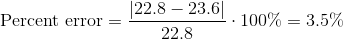### Example Question #71 : High School Chemistry

Joseph calculated that the concentration of an acid solution was 13.2M. The actual concentration of the solution was 16.3M. What is Joseph's percent error?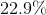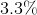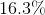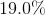Explanation:

Use the following formula to find the percent error:For this experiment, our accepted value is the same as the theoretical value.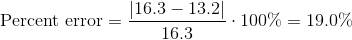### Example Question #72 : High School Chemistry

Patricia measured that the density of a given block of iron was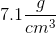. The actual density of iron is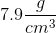. What is Patricia's percent error?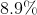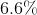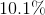Explanation:

Use the following formula to find the percent error:For this experiment, our accepted value is the same as the theoretical value.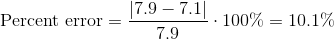### Example Question #11 : Calculating Error

Steven calculated that the melting point of tin is 462 degrees Fahrenheit. The actual melting point of tin is 450 degrees Fahrenheit. What is Steven's percent error?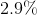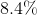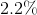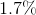Explanation:

Use the following formula to find the percent error:For this experiment, our accepted value is the same as the theoretical value.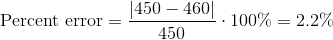### Example Question #11 : Precision, Accuracy, And Error

Through experimentation, Luke found the boiling point of ethanol to be 143 degrees Fahrenheit. The actual boiling point of ethanol is 173 degrees Fahrenheit. What is Luke's percent error?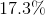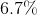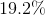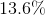Explanation:

Use the following formula to find the percent error:For this experiment, our accepted value is the same as the theoretical value.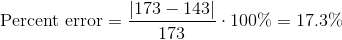### Example Question #81 : High School Chemistry

After conducting an experiment, Kevin produced 56.2 kg of sulfur dioxide gas. However, he was supposed to have been able to produce 84.0 kg of sulfur dioxide gas. What was his percent error?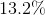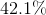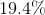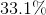Explanation:

Use the following formula to find the percent error:For this experiment, our accepted value is the same as the theoretical value.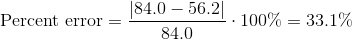### Example Question #81 : High School Chemistry

At the beginning of the experiment, Jimmy calculated an expected yield of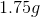of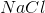. At the end of the experiment, he only recovered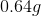. Calculate his percent error.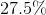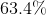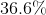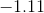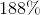Explanation:

Percent error is a calculation usually completed at the end of an experiment for data analysis. It is in essence a tool that can be used to calculate the precision of your calculations. This can be done by following the equation: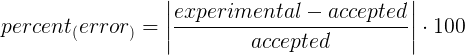Experimental represents that value that was experimentally obtained. Accepted represents that value that would have been accepted.

This quickly turns into a "plug and chug" type of problem.

For this problem:

experimental:accepted: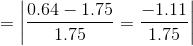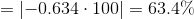### Example Question #82 : High School Chemistry

In the following reaction, eight moles of sodium hydroxide is broken down into four moles of sodium oxide and four moles of water. What is the percent error if your experiment yields 195 grams of sodium oxide?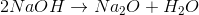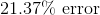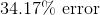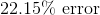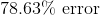Explanation:

To find the percent error we need to use the following equation: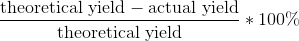But in order to do this, we first have to convert moles of sodium oxide into grams: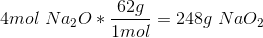This gives us a theoretical yield of 248g, which we plug in with our 195g actual yield.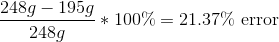### Example Question #83 : High School Chemistry

In the following reaction, eight moles of sodium hydroxide is broken down into four moles of sodium oxide and four moles of water. What is the percent error if your experiment yields 195 grams of sodium oxide?Explanation:

To find the percent error we need to use the following equation:But in order to do this, we first have to convert moles of sodium oxide into grams:This gives us a theoretical yield of 248g, which we plug in with our 195g actual yield.2 Next →

### All High School Chemistry Resources